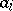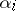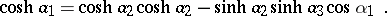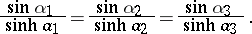# Hyperbolic trigonometry

(diff) ← Older revision | Latest revision (diff) | Newer revision → (diff)

The trigonometry on the Lobachevskii plane (cf. Lobachevskii geometry). Let,, be the lengths of the sides of a triangle on the Lobachevskii plane, and letbe the angles of this triangle. The following relationship (the cosine theorem), which relateswith, is valid:All the remaining relations of hyperbolic trigonometry follow from this one, such as the so-called sine theorem: